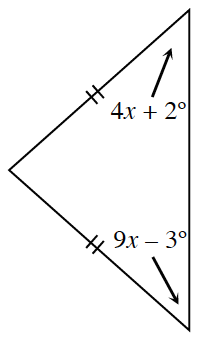### Home > INT2 > Chapter 7 > Lesson 7.2.3 > Problem7-110

7-110.

Multiple Choice: Based on the relationships provided in the diagram, which of the equations below is correct? Justify your solution.

1. $4x+2^\circ+9x-3^\circ=90^\circ$

1. $4x+2^\circ=9x-3^\circ$

1. $4x+2^\circ+9x-3^\circ=180^\circ$

1. $(4x+2^\circ)(9x-3^\circ)=90^\circ$This is an isosceles triangle, because it has two equal side lengths.
So remember in isosceles triangles base angles are equal.